Search this API

## y.layout Class LayoutTool

```java.lang.Objecty.layout.LayoutTool
```

`public class LayoutToolextends java.lang.Object`

The `LayoutTool` provides several helper and utility functions for `LayoutGraph` and the `layout algorithms`.

`LayoutGraph`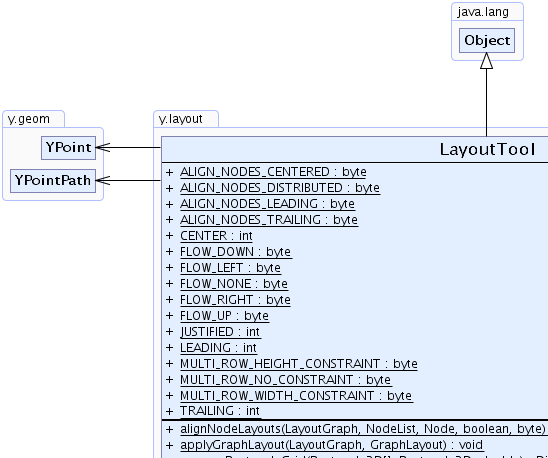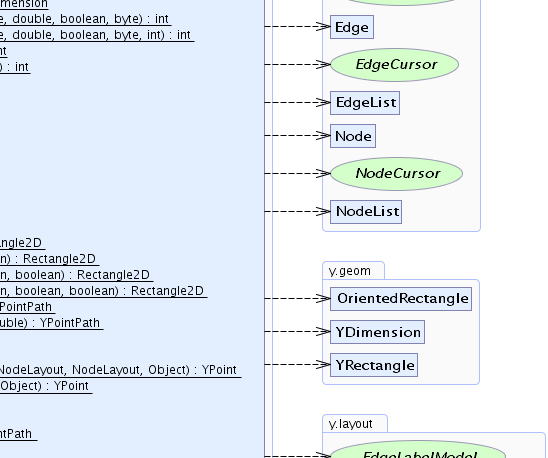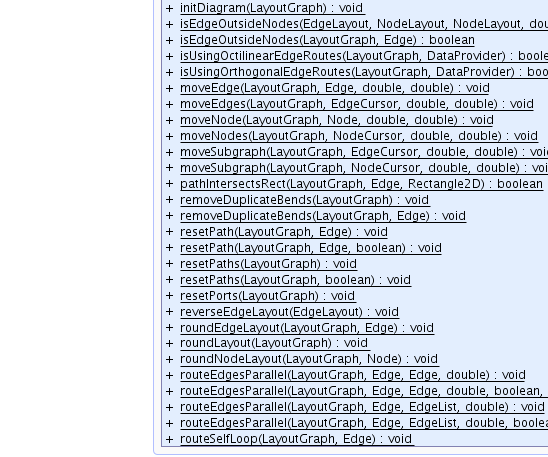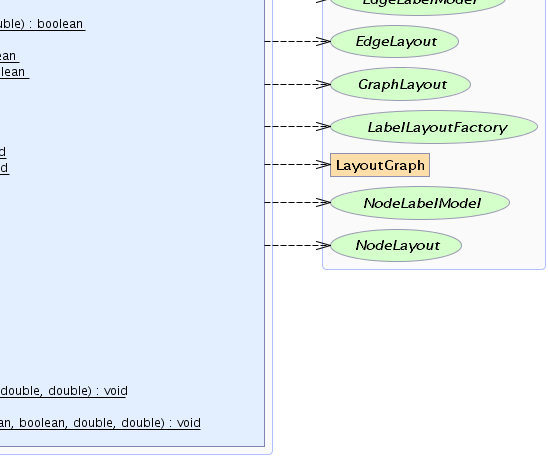Field Summary
`static byte` `ALIGN_NODES_CENTERED`
Node alignment policy that results in center-aligned nodes.
`static byte` `ALIGN_NODES_DISTRIBUTED`
Node alignment policy that results in vertically or horizontally distributed nodes.
`static byte` `ALIGN_NODES_LEADING`
Node alignment policy that results in top-aligned nodes for vertical alignment and left-aligned nodes for horizontal alignments.
`static byte` `ALIGN_NODES_TRAILING`
Node alignment policy that results in bottom-aligned nodes for vertical alignment and right-aligned nodes for horizontal alignments.
`static int` `CENTER`
Row alignment specifier which defines that each row will be aligned with its center.
`static byte` `FLOW_DOWN`
Edge flow specifier which defines that the flow direction of most edges is down.
`static byte` `FLOW_LEFT`
Edge flow specifier which defines that the flow direction of most edges is left.
`static byte` `FLOW_NONE`
Edge flow specifier that defines that there is no main flow direction.
`static byte` `FLOW_RIGHT`
Edge flow specifier which defines that the flow direction of most edges is right.
`static byte` `FLOW_UP`
Edge flow specifier which defines that the flow direction of most edges is up.
`static int` `JUSTIFIED`
Row alignment specifier which defines that each row will be aligned with its left border and its right border.
`static int` `LEADING`
Row alignment specifier which defines that each row will be aligned with its left border.
`static byte` `MULTI_ROW_HEIGHT_CONSTRAINT`
Constraint specifier for a multi-row rectangle arrangement that constrains the height of the layout.
`static byte` `MULTI_ROW_NO_CONSTRAINT`
Constraint specifier for a multi-row rectangle arrangement that is unconstrained.
`static byte` `MULTI_ROW_WIDTH_CONSTRAINT`
Constraint specifier for a multi-row rectangle arrangement that constrains the width of the layout.
`static int` `TRAILING`
Row alignment specifier which defines that each row will be aligned with its right border.

Method Summary
`static void` ```alignNodeLayouts(LayoutGraph graph, NodeList nodes, Node referenceNode, boolean vertical, byte mode)```
Aligns the specified nodes either vertically or horizontally according to the specified node alignment mode.
`static void` ```applyGraphLayout(LayoutGraph graph, GraphLayout layout)```
Applies the layout information in the given `GraphLayout` to the `LayoutGraph`.
`static java.awt.Dimension` ```arrangeRectangleGrid(java.awt.geom.Rectangle2D[] rectangles, java.awt.geom.Rectangle2D finalRect, double aspectRatio)```
Reassigns the bounds of the rectangles in a grid to fit in a large rectangle with an aspect ratio close to the specified aspect ratio.
`static int` ```arrangeRectangleMultiRows(java.awt.geom.Rectangle2D[] rectangles, java.awt.geom.Rectangle2D finalRect, double preferredWidth, double preferredHeight, boolean compaction, byte constraintMode)```
Reassigns the bounds of the rectangles in multiple rows to fit the preferred width and height.
`static int` ```arrangeRectangleMultiRows(java.awt.geom.Rectangle2D[] rectangles, java.awt.geom.Rectangle2D finalRect, double preferredWidth, double preferredHeight, boolean compaction, byte constraintMode, int alignment)```
Reassigns the bounds of the rectangles in multiple rows to fit the preferred width and height.
`static int` ```arrangeRectangleRows(java.awt.geom.Rectangle2D[] rectangles, java.awt.geom.Rectangle2D finalRect, double aspectRatio)```
Reassigns the bounds of the rectangles to fit in a large rectangle with an aspect ratio close to the specified aspect ratio.
`static int` ```arrangeRectangleRows(java.awt.geom.Rectangle2D[] rectangles, java.awt.geom.Rectangle2D finalRect, double aspectRatio, int alignment)```
Reassigns the bounds of the rectangles to fit in a large rectangle with an aspect ratio close to the specified aspect ratio.
`static void` ```assignReverseLayout(LayoutGraph graph, Edge edge1, Edge edge2)```
Applies the reversed layout of the first edge to the second edge.
`static boolean` `autoFlipBox(OrientedRectangle rect)`
Flips the orientation of a given rectangle, if its up vector points downward `(upY > 0)`.
`static void` ```clipEdgeOnBB(LayoutGraph graph, Edge edge)```
Clips the path of the given edge on the bounding box of the source and target points.
`static void` `clipEdgesOnBB(LayoutGraph graph)`
Clips the path of all edges in the graph on the bounding box of their source and target points.
`static byte` ```determineEdgeFlowDirection(LayoutGraph graph, DataProvider considerEdges)```
Determines the main direction of the edge flow by analyzing the current layout of the graph.
`static java.lang.String` ```edgeLayoutString(LayoutGraph graph, Edge edge)```
Returns a `String` representation of the path of the given edge.
`static java.awt.geom.Rectangle2D` ```getBoundingBox(LayoutGraph graph, Edge edge)```
Returns the bounding box of the given edge.
`static java.awt.geom.Rectangle2D` ```getBoundingBox(LayoutGraph graph, EdgeCursor edges)```
Returns the bounding box of the edges accessible through the given cursor.
`static java.awt.geom.Rectangle2D` ```getBoundingBox(LayoutGraph graph, Node node)```
Returns the bounding box of the given node.
`static java.awt.geom.Rectangle2D` ```getBoundingBox(LayoutGraph graph, NodeCursor nodes)```
Returns the bounding box of the nodes accessible through the given cursor.
`static java.awt.geom.Rectangle2D` ```getBoundingBox(LayoutGraph graph, NodeCursor nodes, EdgeCursor edges)```
Returns the bounding box of the nodes and edges accessible through the given cursors.
`static java.awt.geom.Rectangle2D` ```getBoundingBox(LayoutGraph graph, NodeCursor nodes, EdgeCursor edges, boolean includeLabels)```
Returns the bounding box of the nodes and edges accessible through the given cursors, optionally including node labels and edge labels.
`static java.awt.geom.Rectangle2D` ```getBoundingBox(LayoutGraph graph, NodeCursor nodes, EdgeCursor edges, boolean includeNodeLabels, boolean includeEdgeLabels)```
Returns the bounding box of the nodes and edges accessible through the given cursors, optionally including node labels or edge labels.
`static java.awt.geom.Rectangle2D` ```getBoundingBox(LayoutGraph graph, NodeCursor nodes, EdgeCursor edges, boolean includeNodeLabels, boolean includeEdgeLabels, boolean includeHalos)```
Returns the bounding box of the nodes and edges accessible through the given cursors, optionally including node labels, edge labels or `NodeHalo`s.
`static YPointPath` ```getEdgeClippedOnBB(EdgeLayout edge, NodeLayout source, NodeLayout target)```
Returns the path of an edge clipped on the bounding box of the source and target node.
`static YPointPath` ```getEdgeClippedOnBB(EdgeLayout edge, NodeLayout source, NodeLayout target, double inset)```
Returns the path of an edge clipped on insets of the bounding box of the source and target node.
`static YPointPath` ```getEdgeClippedOnBB(LayoutGraph graph, Edge edge)```
Returns the path of an edge clipped on the bounding box of the source and target node.
`static LabelLayoutFactory` `getLabelFactory(LayoutGraph graph)`
Returns the `LabelLayoutFactory` for the given graph.
`static YPoint` ```getLabelPlacement(EdgeLabelModel model, YDimension labelSize, EdgeLayout edgeLayout, NodeLayout sourceLayout, NodeLayout targetLayout, java.lang.Object param)```
Returns the upper-left corner of the paraxial bounding box of the given edge label as encoded by the specified model parameter.
`static YPoint` ```getLabelPlacement(NodeLabelModel model, YDimension labelSize, NodeLayout nodeLayout, java.lang.Object param)```
Returns the upper-left corner of the paraxial bounding box of the given node label as encoded by the specified model parameter.
`static double` ```getNodeDistance(LayoutGraph graph, Node node1, Node node2)```
Returns the distance between the centers of the two given nodes.
`static java.lang.String` `getNodePositions(LayoutGraph graph)`
Returns a `String` representation of all node positions in the graph.
`static YPointPath` ```getPathClippedOnBB(YPointPath path, YRectangle sourceBox, YRectangle targetBox)```
Returns the path of a given edge path clipped on the given bounding boxes of the source and target node.
`static double` ```getPathLength(LayoutGraph graph, Edge edge)```
Returns the length of the path of the given edge.
`static void` `initDiagram(LayoutGraph graph)`
Initializes the given graph.
`static boolean` ```isEdgeOutsideNodes(EdgeLayout edge, NodeLayout source, NodeLayout target, double halo)```
Checks whether or not the path of an edge is outside the bounding box of the source and target node, considering the given halo.
`static boolean` ```isEdgeOutsideNodes(LayoutGraph graph, Edge edge)```
Checks whether or not the path of an edge is outside the bounding box of the source and target node.
`static boolean` ```isUsingOctilinearEdgeRoutes(LayoutGraph graph, DataProvider considerEdges)```
Determines whether or not most of the edges of the graph are routed octilinear.
`static boolean` ```isUsingOrthogonalEdgeRoutes(LayoutGraph graph, DataProvider considerEdges)```
Determines whether or not most of the edges of the graph are routed orthogonally.
`static void` ```moveEdge(LayoutGraph graph, Edge edge, double dx, double dy)```
Moves all control points of the given edge by the vector `(dx,dy)`.
`static void` ```moveEdges(LayoutGraph graph, EdgeCursor edges, double dx, double dy)```
Moves the control points of all edges accessible through the given `EdgeCursor` by the vector `(dx,dy)`.
`static void` ```moveNode(LayoutGraph graph, Node node, double dx, double dy)```
Moves the given node by the vector `(dx,dy)`.
`static void` ```moveNodes(LayoutGraph graph, NodeCursor nodes, double dx, double dy)```
Moves all nodes accessible through the given `NodeCursor` by the vector `(dx,dy)`.
`static void` ```moveSubgraph(LayoutGraph graph, EdgeCursor edges, double dx, double dy)```
Moves the subgraph induced by the edges accessible through the given `EdgeCursor` by the vector `(dx,dy)`.
`static void` ```moveSubgraph(LayoutGraph graph, NodeCursor nodes, double dx, double dy)```
Moves the subgraph induced by the nodes accessible through the given `NodeCursor` by the vector `(dx,dy)`.
`static boolean` ```pathIntersectsRect(LayoutGraph graph, Edge edge, java.awt.geom.Rectangle2D rect)```
Checks whether or not the path of the given edge intersects the interior of a given rectangular area.
`static void` `removeDuplicateBends(LayoutGraph graph)`
Removes successive bends that have the same coordinates from all edges in the graph.
`static void` ```removeDuplicateBends(LayoutGraph graph, Edge edge)```
Removes successive bends that have the same coordinates from the given edge.
`static void` ```resetPath(LayoutGraph graph, Edge edge)```
Assigns a trivial path which has no control points to the specified edge and sets the edge's ports to the center of the corresponding nodes.
`static void` ```resetPath(LayoutGraph graph, Edge edge, boolean resetPorts)```
Assigns a trivial path which has no control points to the specified edge.
`static void` `resetPaths(LayoutGraph graph)`
Assigns a trivial path which has no control points to all edges in the specified graph and sets the edges' ports to the center of the corresponding nodes.
`static void` ```resetPaths(LayoutGraph graph, boolean resetPorts)```
Assigns a trivial path which has no control points to all edges in the specified graph.
`static void` `resetPorts(LayoutGraph graph)`
Sets the ports of all edges in the graph to the center of the nodes.
`static void` `reverseEdgeLayout(EdgeLayout el)`
Reverses the layout of an edge.
`static void` ```roundEdgeLayout(LayoutGraph graph, Edge edge)```
Rounds the locations of the control points and ports of the given edge to integer values.
`static void` `roundLayout(LayoutGraph graph)`
Rounds coordinates and sizes in the layout of an entire graph to integer values.
`static void` ```roundNodeLayout(LayoutGraph graph, Node node)```
Rounds the location and size of the given node to integer values.
`static void` ```routeEdgesParallel(LayoutGraph graph, Edge edge1, Edge edge2, double lineDistance)```
Routes two edges which are incident to the same nodes in parallel.
`static void` ```routeEdgesParallel(LayoutGraph graph, Edge edge1, Edge edge2, double lineDistance, boolean joinEnds, double absJoinDist, double relJoinDist)```
Routes two edges which are incident to the same nodes in parallel, optionally joining the end points.
`static void` ```routeEdgesParallel(LayoutGraph graph, Edge edge, EdgeList edges, double lineDistance)```
Routes a list of edges which are incident to the same nodes in parallel.
`static void` ```routeEdgesParallel(LayoutGraph graph, Edge edge, EdgeList edges, double lineDistance, boolean adjustE1, boolean joinEnds, double absJoinDist, double relJoinDist)```
Routes a list of edges which are incident to the same nodes in parallel, optionally joining the end points.
`static void` ```routeSelfLoop(LayoutGraph graph, Edge edge)```
Routes a self-loop.

Methods inherited from class java.lang.Object
`clone, equals, finalize, getClass, hashCode, notify, notifyAll, toString, wait, wait, wait`

Field Detail

`public static final int LEADING`
Row alignment specifier which defines that each row will be aligned with its left border.

`arrangeRectangleRows(Rectangle2D[], Rectangle2D, double, int)`, Constant Field Values
Sample Graph: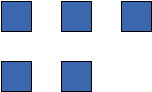### TRAILING

`public static final int TRAILING`
Row alignment specifier which defines that each row will be aligned with its right border.

`arrangeRectangleRows(Rectangle2D[], Rectangle2D, double, int)`, Constant Field Values
Sample Graph:### JUSTIFIED

`public static final int JUSTIFIED`
Row alignment specifier which defines that each row will be aligned with its left border and its right border.

Rectangles in smaller rows will be distributed over the row, so that the row aligns with both sides.

`arrangeRectangleRows(Rectangle2D[], Rectangle2D, double, int)`, Constant Field Values
Sample Graph:### CENTER

`public static final int CENTER`
Row alignment specifier which defines that each row will be aligned with its center.

`arrangeRectangleRows(Rectangle2D[], Rectangle2D, double, int)`, Constant Field Values
Sample Graph: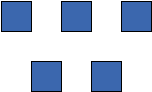### MULTI_ROW_NO_CONSTRAINT

`public static final byte MULTI_ROW_NO_CONSTRAINT`
Constraint specifier for a multi-row rectangle arrangement that is unconstrained. Both, height and width, are not limited in size. The rectangles will be arranged in a way that the view ratio defined by the preferred height and width will be achieved.

`arrangeRectangleMultiRows(Rectangle2D[], Rectangle2D, double, double, boolean, byte, int)`, Constant Field Values

### MULTI_ROW_HEIGHT_CONSTRAINT

`public static final byte MULTI_ROW_HEIGHT_CONSTRAINT`
Constraint specifier for a multi-row rectangle arrangement that constrains the height of the layout. Limits the height of the arrangement to the given preferred height value. If some rectangles are higher than allowed, then they are placed nevertheless, but the constraint will still be considered for the rest and not weakened to the height of the higher rectangles. Having one rectangle being higher than the constraint does always result in arrangements consisting only of a single top-level row.

`arrangeRectangleMultiRows(Rectangle2D[], Rectangle2D, double, double, boolean, byte, int)`, Constant Field Values

### MULTI_ROW_WIDTH_CONSTRAINT

`public static final byte MULTI_ROW_WIDTH_CONSTRAINT`
Constraint specifier for a multi-row rectangle arrangement that constrains the width of the layout. Limits the width of the arrangement to the given preferred width value. If a rectangle is wider than allowed, then it will nevertheless be placed (in a row containing the rectangle alone), but the constraint will be still considered for other rows and not weakened.

`arrangeRectangleMultiRows(Rectangle2D[], Rectangle2D, double, double, boolean, byte, int)`, Constant Field Values

### FLOW_UP

`public static final byte FLOW_UP`
Edge flow specifier which defines that the flow direction of most edges is up.

`determineEdgeFlowDirection(LayoutGraph, DataProvider)`, Constant Field Values

### FLOW_DOWN

`public static final byte FLOW_DOWN`
Edge flow specifier which defines that the flow direction of most edges is down.

`determineEdgeFlowDirection(LayoutGraph, DataProvider)`, Constant Field Values

### FLOW_LEFT

`public static final byte FLOW_LEFT`
Edge flow specifier which defines that the flow direction of most edges is left.

`determineEdgeFlowDirection(LayoutGraph, DataProvider)`, Constant Field Values

### FLOW_RIGHT

`public static final byte FLOW_RIGHT`
Edge flow specifier which defines that the flow direction of most edges is right.

`determineEdgeFlowDirection(LayoutGraph, DataProvider)`, Constant Field Values

### FLOW_NONE

`public static final byte FLOW_NONE`
Edge flow specifier that defines that there is no main flow direction.

`determineEdgeFlowDirection(LayoutGraph, DataProvider)`, Constant Field Values

`public static final byte ALIGN_NODES_LEADING`
Node alignment policy that results in top-aligned nodes for vertical alignment and left-aligned nodes for horizontal alignments.

`alignNodeLayouts(LayoutGraph, NodeList, Node, boolean, byte)`, Constant Field Values
Sample Graph:### ALIGN_NODES_CENTERED

`public static final byte ALIGN_NODES_CENTERED`
Node alignment policy that results in center-aligned nodes.

`alignNodeLayouts(LayoutGraph, NodeList, Node, boolean, byte)`, Constant Field Values
Sample Graph:### ALIGN_NODES_TRAILING

`public static final byte ALIGN_NODES_TRAILING`
Node alignment policy that results in bottom-aligned nodes for vertical alignment and right-aligned nodes for horizontal alignments.

`alignNodeLayouts(LayoutGraph, NodeList, Node, boolean, byte)`, Constant Field Values
Sample Graph: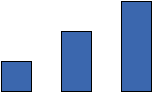### ALIGN_NODES_DISTRIBUTED

`public static final byte ALIGN_NODES_DISTRIBUTED`
Node alignment policy that results in vertically or horizontally distributed nodes. In this context, distributed means that the vertical (or horizontal) extensions of nodes do not overlap, independent of their horizontal (or vertical) position.

`alignNodeLayouts(LayoutGraph, NodeList, Node, boolean, byte)`, Constant Field Values
Sample Graph: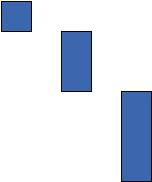Method Detail

### getLabelFactory

`public static LabelLayoutFactory getLabelFactory(LayoutGraph graph)`
Returns the `LabelLayoutFactory` for the given graph.

The factory instance is bound to the specified graph instance, i.e., you can only create, add and remove labels for this graph.
Parameters:
`graph` - the graph
Returns:
the label factory of the graph

### determineEdgeFlowDirection

```public static byte determineEdgeFlowDirection(LayoutGraph graph,
DataProvider considerEdges)```
Determines the main direction of the edge flow by analyzing the current layout of the graph. The method only considers edges that are marked in the given `DataProvider`.

Parameters:
`graph` - the underlying graph
`considerEdges` - the edges to consider
Returns:
one of the predefined flow directions

### isUsingOrthogonalEdgeRoutes

```public static boolean isUsingOrthogonalEdgeRoutes(LayoutGraph graph,
DataProvider considerEdges)```
Determines whether or not most of the edges of the graph are routed orthogonally. The method only considers edges marked in the given `DataProvider`.

Parameters:
`graph` - the underlying graph
`considerEdges` - the edges to consider
Returns:
`true` if most of the edges in the graph are routed orthogonally, `false` otherwise

### isUsingOctilinearEdgeRoutes

```public static boolean isUsingOctilinearEdgeRoutes(LayoutGraph graph,
DataProvider considerEdges)```
Determines whether or not most of the edges of the graph are routed octilinear. The method only considers edges marked in the given `DataProvider`.

This method also returns `true` for orthogonal layouts.
Parameters:
`graph` - the underlying graph
`considerEdges` - the edges to consider
Returns:
`true` if most of the edges in the graph are routed octilinear, `false` otherwise

### getNodeDistance

```public static double getNodeDistance(LayoutGraph graph,
Node node1,
Node node2)```
Returns the distance between the centers of the two given nodes.

Parameters:
`graph` - the graph to which the nodes belong
`node1` - the first node
`node2` - the second node
Returns:
the distance between the nodes' centers

### getPathLength

```public static double getPathLength(LayoutGraph graph,
Edge edge)```
Returns the length of the path of the given edge. The path starts at the source point, traverses through all control points and ends at the target point.

Parameters:
`graph` - the graph to which the edge belongs
`edge` - the edge
Returns:
the length of the edge's path

### reverseEdgeLayout

`public static void reverseEdgeLayout(EdgeLayout el)`
Reverses the layout of an edge. The order of the control points is reversed and source and target point are swapped.

This method will not reverse the edge in the graph structure.
Parameters:
`el` - the layout information of an edge

### resetPaths

`public static void resetPaths(LayoutGraph graph)`
Assigns a trivial path which has no control points to all edges in the specified graph and sets the edges' ports to the center of the corresponding nodes.

Calling this method is equivalent to `resetPaths(LayoutGraph, boolean)` resetting the ports.
Parameters:
`graph` - the graph
`resetPaths(LayoutGraph, boolean)`

### resetPaths

```public static void resetPaths(LayoutGraph graph,
boolean resetPorts)```
Assigns a trivial path which has no control points to all edges in the specified graph.

Parameters:
`graph` - the graph
`resetPorts` - `true` if the ports of the edges should be set to the center of the corresponding nodes, `false` otherwise

### resetPath

```public static void resetPath(LayoutGraph graph,
Edge edge)```
Assigns a trivial path which has no control points to the specified edge and sets the edge's ports to the center of the corresponding nodes.

Calling this method is equivalent to `resetPath(LayoutGraph, Edge, boolean)` resetting the ports.
Parameters:
`graph` - the graph
`edge` - the edge
`resetPath(LayoutGraph, Edge, boolean)`

### resetPath

```public static void resetPath(LayoutGraph graph,
Edge edge,
boolean resetPorts)```
Assigns a trivial path which has no control points to the specified edge.

Parameters:
`graph` - the graph
`edge` - the edge
`resetPorts` - `true` if the ports of the edge should be set to the center of the corresponding nodes, `false` otherwise

### removeDuplicateBends

`public static void removeDuplicateBends(LayoutGraph graph)`
Removes successive bends that have the same coordinates from all edges in the graph.

Parameters:
`graph` - the graph

### removeDuplicateBends

```public static void removeDuplicateBends(LayoutGraph graph,
Edge edge)```
Removes successive bends that have the same coordinates from the given edge.

Parameters:
`graph` - the graph
`edge` - the edge

### moveEdge

```public static void moveEdge(LayoutGraph graph,
Edge edge,
double dx,
double dy)```
Moves all control points of the given edge by the vector `(dx,dy)`.

Parameters:
`graph` - the graph
`edge` - the edge
`dx` - the horizontal distance to move
`dy` - the vertical distance to move

### moveEdges

```public static void moveEdges(LayoutGraph graph,
EdgeCursor edges,
double dx,
double dy)```
Moves the control points of all edges accessible through the given `EdgeCursor` by the vector `(dx,dy)`.

Parameters:
`graph` - the graph
`edges` - the edges to be moved
`dx` - the horizontal distance to move
`dy` - the vertical distance to move

### moveNode

```public static void moveNode(LayoutGraph graph,
Node node,
double dx,
double dy)```
Moves the given node by the vector `(dx,dy)`.

Parameters:
`graph` - the graph
`node` - the node
`dx` - the horizontal distance to move
`dy` - the vertical distance to move

### moveNodes

```public static void moveNodes(LayoutGraph graph,
NodeCursor nodes,
double dx,
double dy)```
Moves all nodes accessible through the given `NodeCursor` by the vector `(dx,dy)`.

Parameters:
`graph` - the graph
`nodes` - the nodes to be moved
`dx` - the horizontal distance to move
`dy` - the vertical distance to move

### moveSubgraph

```public static void moveSubgraph(LayoutGraph graph,
EdgeCursor edges,
double dx,
double dy)```
Moves the subgraph induced by the edges accessible through the given `EdgeCursor` by the vector `(dx,dy)`.

Parameters:
`graph` - the graph that contains the subgraph
`edges` - the edges that induce the subgraph
`dx` - the horizontal distance to move
`dy` - the vertical distance to move

### moveSubgraph

```public static void moveSubgraph(LayoutGraph graph,
NodeCursor nodes,
double dx,
double dy)```
Moves the subgraph induced by the nodes accessible through the given `NodeCursor` by the vector `(dx,dy)`.

Parameters:
`graph` - the graph that contains the subgraph
`nodes` - the nodes that induce the subgraph
`dx` - the horizontal distance to move
`dy` - the vertical distance to move

### getBoundingBox

```public static java.awt.geom.Rectangle2D getBoundingBox(LayoutGraph graph,
NodeCursor nodes,
EdgeCursor edges)```
Returns the bounding box of the nodes and edges accessible through the given cursors.

In this case, the bounding box is the smallest paraxial rectangle which contains the given nodes and the control points as well as the source and target points of all edges.

The bounding box does not include node labels or edge labels.
Parameters:
`graph` - the graph that contains the nodes and edges
`nodes` - the nodes to include in the bounding box
`edges` - the edges to include in the bounding box
Returns:
the bounding box containing the given nodes and edges
`getBoundingBox(LayoutGraph, EdgeCursor)`, `getBoundingBox(LayoutGraph, NodeCursor)`

### getBoundingBox

```public static java.awt.geom.Rectangle2D getBoundingBox(LayoutGraph graph,
Node node)```
Returns the bounding box of the given node.

In this case, the bounding box is the smallest paraxial rectangle which contains the given node. It is identical to the node's bounds.

The bounding box does not include node labels.
Parameters:
`graph` - the graph that contains the node
`node` - the node
Returns:
the bounding box of the node
`LayoutGraph.getNodeLayout(Object)`

### getBoundingBox

```public static java.awt.geom.Rectangle2D getBoundingBox(LayoutGraph graph,
NodeCursor nodes)```
Returns the bounding box of the nodes accessible through the given cursor.

In this case, the bounding box is the smallest paraxial rectangle which contains the given nodes.

The bounding box does not include node labels.
Parameters:
`graph` - the graph that contains the nodes
`nodes` - the nodes to include in the bounding box
Returns:
the bounding box containing the given nodes
`LayoutGraph.getNodeLayout(Object)`

### getBoundingBox

```public static java.awt.geom.Rectangle2D getBoundingBox(LayoutGraph graph,
Edge edge)```
Returns the bounding box of the given edge.

In this case, the bounding box is the smallest paraxial rectangle which contains the control points as well as the absolute coordinates of the source and target point of the edge.

The bounding box does not include edge labels.
Parameters:
`graph` - the graph that contains the edge
`edge` - the edge
Returns:
the bounding box of the edge
`LayoutGraph.getSourcePointAbs(Edge)`, `LayoutGraph.getTargetPointAbs(Edge)`, `LayoutGraph.getLayout(Edge)`

### getBoundingBox

```public static java.awt.geom.Rectangle2D getBoundingBox(LayoutGraph graph,
EdgeCursor edges)```
Returns the bounding box of the edges accessible through the given cursor.

In this case, the bounding box is the smallest paraxial rectangle which contains the control points as well as the absolute coordinates of the source and target points of all given edges.

The bounding box does not include edge labels.
Parameters:
`graph` - the graph that contains the edges
`edges` - the edges to include in the bounding box
Returns:
the bounding box containing the given edges
`LayoutGraph.getSourcePointAbs(Edge)`, `LayoutGraph.getTargetPointAbs(Edge)`, `LayoutGraph.getLayout(Edge)`

### getBoundingBox

```public static java.awt.geom.Rectangle2D getBoundingBox(LayoutGraph graph,
NodeCursor nodes,
EdgeCursor edges,
boolean includeLabels)```
Returns the bounding box of the nodes and edges accessible through the given cursors, optionally including node labels and edge labels.

In this case, the bounding box is the smallest paraxial rectangle which contains the given nodes and the control points as well as the source and target points of all edges. If labels should be included, it also contains their boxes.

Parameters:
`graph` - the graph that contains the given nodes and edges
`nodes` - the nodes to include in the bounding box
`edges` - the edges to include in the bounding box
`includeLabels` - `true` if labels of nodes and edges should be included, `false` otherwise
Returns:
the bounding box of the given nodes and edges
`getBoundingBox(LayoutGraph, NodeCursor, EdgeCursor, boolean, boolean)`

### getBoundingBox

```public static java.awt.geom.Rectangle2D getBoundingBox(LayoutGraph graph,
NodeCursor nodes,
EdgeCursor edges,
boolean includeNodeLabels,
boolean includeEdgeLabels)```
Returns the bounding box of the nodes and edges accessible through the given cursors, optionally including node labels or edge labels.

In this case, the bounding box is the smallest paraxial rectangle which contains the given nodes and the control points as well as the source and target points of all edges. If node or edge labels should be included, it also contains their boxes.

Parameters:
`graph` - the graph that contains the given nodes and edges
`nodes` - the nodes to include in the bounding box
`edges` - the edges to include in the bounding box
`includeNodeLabels` - `true` if labels of nodes should be included, `false` otherwise
`includeEdgeLabels` - `true` if labels of edges should be included, `false` otherwise
Returns:
the bounding box of the given nodes and edges

### getBoundingBox

```public static java.awt.geom.Rectangle2D getBoundingBox(LayoutGraph graph,
NodeCursor nodes,
EdgeCursor edges,
boolean includeNodeLabels,
boolean includeEdgeLabels,
boolean includeHalos)```
Returns the bounding box of the nodes and edges accessible through the given cursors, optionally including node labels, edge labels or `NodeHalo`s.

In this case, the bounding box is the smallest paraxial rectangle which contains the given nodes and the control points as well as the source and target points of all edges. If node or edge labels should be included, it also contains their boxes. `NodeHalo`s can also extend the bounding box if desired.

Parameters:
`graph` - the graph that contains the given nodes and edges
`nodes` - the nodes to include in the bounding box
`edges` - the edges to include in the bounding box
`includeNodeLabels` - `true` if labels of nodes should be included, `false` otherwise
`includeEdgeLabels` - `true` if labels of edges should be included, `false` otherwise
`includeHalos` - `true` if `NodeHalo`s should be included, `false` otherwise
Returns:
the bounding box of the given nodes and edges

### getLabelPlacement

```public static YPoint getLabelPlacement(NodeLabelModel model,
YDimension labelSize,
NodeLayout nodeLayout,
java.lang.Object param)```
Returns the upper-left corner of the paraxial bounding box of the given node label as encoded by the specified model parameter.

The parameter describes the abstract position of the label within the specified model. The parameter must have been generated by that model.

Parameters:
`model` - the `NodeLabelModel` used for placing the label
`labelSize` - the size of the label
`nodeLayout` - the layout of the node to which the label belongs
`param` - the model parameter
Returns:
the upper-left corner of the label's bounding box
`NodeLabelModel.getLabelPlacement(YDimension, NodeLayout, Object)`, `OrientedRectangle.getBoundingBox()`

### getLabelPlacement

```public static YPoint getLabelPlacement(EdgeLabelModel model,
YDimension labelSize,
EdgeLayout edgeLayout,
NodeLayout sourceLayout,
NodeLayout targetLayout,
java.lang.Object param)```
Returns the upper-left corner of the paraxial bounding box of the given edge label as encoded by the specified model parameter.

The parameter describes the abstract position of the label within the specified model. The parameter must have been generated by that model.

Parameters:
`model` - the `EdgeLabelModel` used for placing the label
`labelSize` - the size of the label that should be placed
`edgeLayout` - the layout of the edge to which the label belongs
`sourceLayout` - the layout of the edge's source node
`targetLayout` - the layout of the edge's target node
`param` - the model parameter
Returns:
the upper-left corner of the label's bounding box
`EdgeLabelModel.getLabelPlacement(YDimension, EdgeLayout, NodeLayout, NodeLayout, Object)`, `OrientedRectangle.getBoundingBox()`

### resetPorts

`public static void resetPorts(LayoutGraph graph)`
Sets the ports of all edges in the graph to the center of the nodes.

The control points will not be changed.
Parameters:
`graph` - the graph
`LayoutGraph.setSourcePointRel(Edge, YPoint)`, `LayoutGraph.setTargetPointRel(Edge, YPoint)`

### getEdgeClippedOnBB

```public static YPointPath getEdgeClippedOnBB(EdgeLayout edge,
NodeLayout source,
NodeLayout target)```
Returns the path of an edge clipped on the bounding box of the source and target node.

On the clipped edge, the source and target port will be located at the border of the corresponding node.

If the source/target end of the edge's path is not contained in the specified source/target node layout, the algorithm just keeps that end of the path as it is, i.e., this method does never extend the path.
Parameters:
`edge` - the clipped edge
`source` - the source of the edge
`target` - the target of the edge
Returns:
the clipped path of the edge

### getEdgeClippedOnBB

```public static YPointPath getEdgeClippedOnBB(LayoutGraph graph,
Edge edge)```
Returns the path of an edge clipped on the bounding box of the source and target node.

On the clipped edge, the source and target port will be located at the border of the corresponding node.

If the source/target end of the edge's path is not contained in the source/target node layout, the algorithm just keeps that end of the path as it is, i.e., this method does never extend the path.
Parameters:
`graph` - the graph to which the edge belongs
`edge` - the clipped edge
Returns:
the clipped path of the edge

### getEdgeClippedOnBB

```public static YPointPath getEdgeClippedOnBB(EdgeLayout edge,
NodeLayout source,
NodeLayout target,
double inset)```
Returns the path of an edge clipped on insets of the bounding box of the source and target node.

On the clipped edge, the source and target port will be located inside the node with an inset to the border of the corresponding node.

If the source/target end of the given path is not contained in the specified source/target node layout, the algorithm just keeps that end of the path as it is, i.e., this method does never extend the path.
Parameters:
`edge` - the clipped edge
`source` - the source of the edge
`target` - the target of the edge
`inset` - the inset of the node
Returns:
the clipped path of the edge

### getPathClippedOnBB

```public static YPointPath getPathClippedOnBB(YPointPath path,
YRectangle sourceBox,
YRectangle targetBox)```
Returns the path of a given edge path clipped on the given bounding boxes of the source and target node.

In contrast to methods `getEdgeClippedOnBB(EdgeLayout, NodeLayout, NodeLayout, double)`, `getEdgeClippedOnBB(LayoutGraph, Edge)` and `getEdgeClippedOnBB(EdgeLayout, NodeLayout, NodeLayout)`, the path can explicitly be specified and is not queried from a given graph and edge. Furthermore, this method does not consider any insets on the given boxes
Parameters:
`path` - the path that will be clipped
`sourceBox` - the box of the source node that the given path should be clipped at
`targetBox` - the box of the target node that the given path should be clipped at
Returns:
the path clipped on the given nodes boxes

### isEdgeOutsideNodes

```public static boolean isEdgeOutsideNodes(LayoutGraph graph,
Edge edge)```
Checks whether or not the path of an edge is outside the bounding box of the source and target node.

The edge is outside the nodes if its control points as well as the source and target point are not inside the nodes' bounding boxes.

Parameters:
`graph` - the graph to which the edge belongs
`edge` - the edge
Returns:
`true` if the edge is outside the nodes, `false` otherwise

### isEdgeOutsideNodes

```public static boolean isEdgeOutsideNodes(EdgeLayout edge,
NodeLayout source,
NodeLayout target,
double halo)```
Checks whether or not the path of an edge is outside the bounding box of the source and target node, considering the given halo.

The edge is outside the nodes if its control points as well as the source and target point are not inside the nodes' bounding boxes.

The nodes can be enlarged by a halo to check if the edge keeps some distance to the nodes.

Parameters:
`edge` - the edge
`source` - the source of the edge
`target` - the target of the edge
`halo` - the halo for the nodes
Returns:
`true` if the edge is outside the nodes, `false` otherwise

### clipEdgesOnBB

`public static void clipEdgesOnBB(LayoutGraph graph)`
Clips the path of all edges in the graph on the bounding box of their source and target points.

On a clipped edge, the source and target port will be located at the border of the corresponding node.

If the source/target end of an edge's path is not contained in the associated source/target node layout, the algorithm just keeps that end of the path as it is, i.e., this method does never extend the path.
Parameters:
`graph` - the graph
`clipEdgeOnBB(LayoutGraph, Edge)`

### clipEdgeOnBB

```public static void clipEdgeOnBB(LayoutGraph graph,
Edge edge)```
Clips the path of the given edge on the bounding box of the source and target points.

On a clipped edge, the source and target port will be located at the border of the corresponding node.

If the source/target end of the edge's path is not contained in the source/target node layout, the algorithm just keeps that end of the path as it is, i.e., this method does never extend the path.
Parameters:
`graph` - the graph to which the edge belongs
`edge` - the edge to clip

### edgeLayoutString

```public static java.lang.String edgeLayoutString(LayoutGraph graph,
Edge edge)```
Returns a `String` representation of the path of the given edge.

The source and target ports are not included in the representation.
Parameters:
`graph` - the graph to which the edge belongs
`edge` - the edge
Returns:
the `String` that contains all control points of the edge

### roundNodeLayout

```public static void roundNodeLayout(LayoutGraph graph,
Node node)```
Rounds the location and size of the given node to integer values.

Parameters:
`graph` - the graph to which the node belongs
`node` - the node whose values are rounded

### roundEdgeLayout

```public static void roundEdgeLayout(LayoutGraph graph,
Edge edge)```
Rounds the locations of the control points and ports of the given edge to integer values.

Parameters:
`graph` - the graph to which the edge belongs
`edge` - the edge whose values are rounded

### roundLayout

`public static void roundLayout(LayoutGraph graph)`
Rounds coordinates and sizes in the layout of an entire graph to integer values.

Parameters:
`graph` - the graph
`roundNodeLayout(LayoutGraph, Node)`, `roundEdgeLayout(LayoutGraph, Edge)`

### routeEdgesParallel

```public static void routeEdgesParallel(LayoutGraph graph,
Edge edge1,
Edge edge2,
double lineDistance)```
Routes two edges which are incident to the same nodes in parallel.

The second edge gets a path that is parallel to the path of the first (leading) edge.

Parameters:
`graph` - the graph
`edge1` - the leading edge
`edge2` - the edge to be adjusted
`lineDistance` - the distance between the two edges
`routeEdgesParallel(LayoutGraph, Edge, Edge, double, boolean, double, double)`

### routeEdgesParallel

```public static void routeEdgesParallel(LayoutGraph graph,
Edge edge1,
Edge edge2,
double lineDistance,
boolean joinEnds,
double absJoinDist,
double relJoinDist)```
Routes two edges which are incident to the same nodes in parallel, optionally joining the end points.

The second edge gets a path that is parallel to the path of the first (leading) edge.

Parameters:
`graph` - the graph
`edge1` - the leading edge
`edge2` - the edge to be adjusted
`lineDistance` - the distance between the two edges
`joinEnds` - `true` if the edges should share their end points (the ones of the leading edge), `false` otherwise
`absJoinDist` - the absolute distance between the end points and the beginning of the parallel segment routing if the ends are joined
`relJoinDist` - the relative distance, measured relative to the length of the first/last segments if the ends are joined
`routeEdgesParallel(LayoutGraph, Edge, EdgeList, double, boolean, boolean, double, double)`

### routeEdgesParallel

```public static void routeEdgesParallel(LayoutGraph graph,
Edge edge,
EdgeList edges,
double lineDistance)```
Routes a list of edges which are incident to the same nodes in parallel.

All edges get a path that is parallel to the path of the first (leading) edge.

Parameters:
`graph` - the graph
`edge` - the leading edge
`edges` - the edges to be adjusted
`lineDistance` - the distance between two edges
`routeEdgesParallel(LayoutGraph, Edge, EdgeList, double, boolean, boolean, double, double)`

### routeEdgesParallel

```public static void routeEdgesParallel(LayoutGraph graph,
Edge edge,
EdgeList edges,
double lineDistance,
boolean joinEnds,
double absJoinDist,
double relJoinDist)```
Routes a list of edges which are incident to the same nodes in parallel, optionally joining the end points.

All edges get a path that is parallel to the path of the first (leading) edge.

The position of the leading edge may be adjusted if there is an odd number of parallel edges. It will be moved from the center to achieve a more symmetric port assignment.

Parameters:
`graph` - the graph
`edge` - the leading edge
`edges` - the list of edges that will be aligned with the leading edge
`lineDistance` - the distance between two edges
`adjustE1` - `true` if the leading edge should be adjusted if the size of list is odd, `false` otherwise
`joinEnds` - `true` if the edges should share their end points (the ones of the leading edge), `false` otherwise
`absJoinDist` - the absolute distance between the end points and the beginning of the parallel segment routing if the ends are joined
`relJoinDist` - the relative distance, measured relative to the length of the first/last segments if the ends are joined

### routeSelfLoop

```public static void routeSelfLoop(LayoutGraph graph,
Edge edge)```
Routes a self-loop.

For a self-loop edge, source and target are identical. The path of the self-loop will start at the top of the node, go around the upper-left corner and end at the left side of the node.

Parameters:
`graph` - the graph to which the edge belongs
`edge` - the self-loop

### assignReverseLayout

```public static void assignReverseLayout(LayoutGraph graph,
Edge edge1,
Edge edge2)```
Applies the reversed layout of the first edge to the second edge.

Parameters:
`graph` - the graph to which both edges belong
`edge1` - the edge which adopts the points
`edge2` - the template

### getNodePositions

`public static java.lang.String getNodePositions(LayoutGraph graph)`
Returns a `String` representation of all node positions in the graph.

Parameters:
`graph` - the graph
Returns:
the `String` containing all node locations
`LayoutGraph.getLocation(Node)`

### initDiagram

`public static void initDiagram(LayoutGraph graph)`
Initializes the given graph.

All nodes are placed at `(0,0)`. The edges get connected to the center of their sources and targets, while all their control points are removed.

Parameters:
`graph` - the graph
`LayoutGraph.setLocation(Node, double, double)`, `resetPaths(LayoutGraph)`

### arrangeRectangleRows

```public static int arrangeRectangleRows(java.awt.geom.Rectangle2D[] rectangles,
java.awt.geom.Rectangle2D finalRect,
double aspectRatio)```
Reassigns the bounds of the rectangles to fit in a large rectangle with an aspect ratio close to the specified aspect ratio. This is achieved by arranging the rectangles in rows.

This method invokes `arrangeRectangleRows` with `LEADING` as the last argument.

The aspect ratio must be greater than `0`.

Parameters:
`rectangles` - the rectangles whose coordinates will be modified
`finalRect` - the exact dimension of the resulting rectangle
`aspectRatio` - the preferred aspect ratio of the resulting rectangle
Returns:
the number of rows
Throws:
`java.lang.IllegalArgumentException` - if the aspect ratio is `0` or less

### arrangeRectangleGrid

```public static java.awt.Dimension arrangeRectangleGrid(java.awt.geom.Rectangle2D[] rectangles,
java.awt.geom.Rectangle2D finalRect,
double aspectRatio)```
Reassigns the bounds of the rectangles in a grid to fit in a large rectangle with an aspect ratio close to the specified aspect ratio.

The aspect ratio must be greater than `0`.

Parameters:
`rectangles` - the rectangles whose coordinates will be modified
`finalRect` - the exact dimension will be placed in this rectangle
`aspectRatio` - the preferred aspect ratio of the grid
Returns:
the dimension of the grid
Throws:
`java.lang.IllegalArgumentException` - if the given aspect ratio is less than or equal to zero

### arrangeRectangleMultiRows

```public static int arrangeRectangleMultiRows(java.awt.geom.Rectangle2D[] rectangles,
java.awt.geom.Rectangle2D finalRect,
double preferredWidth,
double preferredHeight,
boolean compaction,
byte constraintMode)```
Reassigns the bounds of the rectangles in multiple rows to fit the preferred width and height. The rectangles will be placed within this bound, arranged in rows, where each row may be split into more sub-rows. This often leads to more compact results than provided by the procedure used in `arrangeRectangleRows(Rectangle2D[], Rectangle2D, double, int)`. A row will be subdivided into more rows, if two successive rectangles to be placed in the row could be placed stacked within this row without making the row larger in height.

The preferred width and height should be greater than `0`, otherwise the nodes are not arranged.

Parameters:
`rectangles` - the rectangles to arrange (coordinates will be assigned)
`finalRect` - the exact resulting dimension will be stored in this rectangle
`preferredWidth` - the preferred width of the result rectangle
`preferredHeight` - the preferred height of the result rectangle
`compaction` - `true` if the algorithm tries to always minimize the resulting dimension, whilst respecting the given constraints, `false` otherwise
`constraintMode` - the width or height constraints to consider
Returns:
the number of rows used
`arrangeRectangleMultiRows(Rectangle2D[], Rectangle2D, double, double, boolean, byte, int)`

### arrangeRectangleMultiRows

```public static int arrangeRectangleMultiRows(java.awt.geom.Rectangle2D[] rectangles,
java.awt.geom.Rectangle2D finalRect,
double preferredWidth,
double preferredHeight,
boolean compaction,
byte constraintMode,
int alignment)```
Reassigns the bounds of the rectangles in multiple rows to fit the preferred width and height. The rectangles will be placed within this bound, arranged in rows, where each row may be split into more sub-rows. This often leads to more compact results than provided by the procedure used in `arrangeRectangleRows(Rectangle2D[], Rectangle2D, double, int)`. A row will be subdivided into more rows, if two successive rectangles to be placed in the row could be placed stacked within this row without making the row larger in height.

The preferred width and height should be greater than `0`, otherwise the nodes are not arranged.

Parameters:
`rectangles` - the rectangles to arrange (coordinates will be assigned)
`finalRect` - the exact resulting dimension will be stored in this rectangle
`preferredWidth` - the preferred width of the result rectangle
`preferredHeight` - the preferred height of the result rectangle
`compaction` - `true` if the algorithm tries to always minimize the resulting dimension, whilst respecting the given constraints, `false` otherwise
`constraintMode` - the width or height constraints to consider
`alignment` - the alignment inside a row
Returns:
the number of rows used
`arrangeRectangleMultiRows(Rectangle2D[], Rectangle2D, double, double, boolean, byte, int)`

### arrangeRectangleRows

```public static int arrangeRectangleRows(java.awt.geom.Rectangle2D[] rectangles,
java.awt.geom.Rectangle2D finalRect,
double aspectRatio,
int alignment)```
Reassigns the bounds of the rectangles to fit in a large rectangle with an aspect ratio close to the specified aspect ratio. This is achieved by arranging the rectangles in rows.

The aspect ratio must be greater than `0`.

Parameters:
`rectangles` - the rectangles whose coordinates will be modified
`finalRect` - the exact dimension of the resulting rectangle
`aspectRatio` - the preferred aspect ratio of the resulting rectangle
`alignment` - one of the valid row alignments
Returns:
the number of used rows
Throws:
`java.lang.IllegalArgumentException` - if the aspect ratio is `0` or less

### pathIntersectsRect

```public static boolean pathIntersectsRect(LayoutGraph graph,
Edge edge,
java.awt.geom.Rectangle2D rect)```
Checks whether or not the path of the given edge intersects the interior of a given rectangular area.

Parameters:
`graph` - the graph to which the edge belongs
`edge` - the edge
`rect` - the rectangular area to check for intersection
Returns:
`true` if the edge path intersects with the given rectangle, `false` otherwise

### applyGraphLayout

```public static void applyGraphLayout(LayoutGraph graph,
GraphLayout layout)```
Applies the layout information in the given `GraphLayout` to the `LayoutGraph`.

All nodes get coordinates and sizes. The edges get coordinates for the control points. For both nodes and edges the labels are placed.

Precondition:
The `GraphLayout` data must be defined for elements in the given `LayoutGraph`.
Parameters:
`graph` - the graph
`layout` - the layout information for the graph

### alignNodeLayouts

```public static void alignNodeLayouts(LayoutGraph graph,
NodeList nodes,
Node referenceNode,
boolean vertical,
byte mode)```
Aligns the specified nodes either vertically or horizontally according to the specified node alignment mode.

For vertical arrangements, nodes are either `top-aligned`, `center-aligned`, `bottom-aligned`, or `vertically distributed`.

For horizontal arrangements, nodes are either `left-aligned`, `center-aligned`, `right-aligned`, or `horizontally distributed`.

In this context, distributed means that the specified nodes are placed in such a way that the projections of their bounds onto the vertical or horizontal coordinate axis do not overlap. For example, after vertically distributing nodes, `(n1.y + n1.height) <= n2.y` for two consecutively placed nodes `n1` and `n2`.

Parameters:
`graph` - the graph that contains the specified nodes
`nodes` - the subset of the specified graph's nodes that is arranged
`referenceNode` - if `null`, the common coordinate for aligning the specified nodes is calculated as the the vertical minimum (top-aligned), the vertical center, the vertical maximum (bottom-aligned), the horizontal minimum (left-aligned), the horizontal center, or the horizontal maximum (right-aligned) of the bounds of the specified nodes; otherwise the appropriate coordinate of the given reference node's bounds is used as common coordinate for aligning the specified nodes. The reference node is ignored when `distributing` nodes.
`vertical` - `true` if nodes should be aligned (or distributed) according to their y-coordinates; otherwise nodes are aligned (or distributed) according to their x-coordinates.
`mode` - one of the valid alignment modes

### autoFlipBox

`public static boolean autoFlipBox(OrientedRectangle rect)`
Flips the orientation of a given rectangle, if its up vector points downward `(upY > 0)`. That means, it rotates the rectangle by `180` degrees without changing the rectangle's center.

Parameters:
`rect` - the given rectangle
Returns:
`true` if the given rectangle was flipped, `false` otherwise